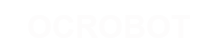#### 站点工具

reference:language:bitshift

# bitshift left (<<), bitshift right (>>)

## 语法

variable « number_of_bits variable » number_of_bits

## 参数

variable - (byte, int, long) number_of_bits integer ⇐ 32

## 例子

```    int a = 5;        // 二进制数: 0000000000000101
int b = a << 3;   // 二进制数: 0000000000101000, 或十进制数：40
int c = b >> 3;   // 二进制数: 0000000000000101, 或者说回到开始时的5```

```    int a = 5;        // 二进制: 0000000000000101
int b = a << 14;  // 二进制: 0100000000000000 - 101中最左边的1被丢弃```

```1 << 0 == 1
1 << 1 == 2
1 << 2 == 4
1 << 3 == 8
...
1 << 8 == 256
1 << 9 == 512
10 << 1 == 1024
...```

```X = -16; //二进制：1111111111110000
Y = X >> 3 //二进制：1111111111111110```

```X = -16; //二进制：1111111111110000
int y = (unsigned int)x >> 3;  // 二进制: 0001111111111110```

```INT = 1000;
Y = X >> 3; 8 1000  //1000整除8，使y=125```
reference/language/bitshift.txt · 最后更改: 2017/04/07 10:03 (外部编辑)

### 页面工具